# KSEEB SSLC Class 10 Maths Solutions Chapter 14 Probability Ex 14.1

In this chapter, we provide KSEEB SSLC Class 10 Maths Solutions Solutions Chapter 14 Probability Ex 14.1 for English medium students, Which will very helpful for every student in their exams. Students can download the latest KSEEB SSLC Class 10 Maths Solutions Solutions Chapter 14 Probability Ex 14.1 pdf, free KSEEB SSLC Class 10 Maths Solutions Solutions Chapter 14 Probability Ex 14.1 pdf download. Now you will get step by step solution to each question.

### Karnataka State Syllabus Class 10 Maths SolutionsChapter 14 Probability Ex 14.1

Question 1.
Complete the following statements :

1. Probability of an event E + Probability of the event ‘not E’ = _____
2. The Probability of an event that cannot happen is _____. Such an event is called ____.
3. The probability of an event that is certain to happen is ____. Such an event is called ____.
4. The sum of the probabilities of all the elementary events of an experiment is ____.
5. The probability of an event is greater than or equal to ____ and less than or equal to ____.

1. 1 : Probability of an event E + Probability of the event ‘not E’ = 1.
2. 0, impossible: The probability of an event that cannot happen is 0. Such an event is called impossible event.
3. 1, certain: The probability of an event that is certain to happen is 1. Such an event is called a sure or certain event.
4. 1: The sum of the probabilities of all the elementary events of an experiment is 1.
5. 0, 1: The probability of an event is greater than or equal to 0 and less than or equal to 1.

Question 2.
Which of the following experiments have equally likely outcomes? Explain.
(i) A driver attempts to start a car. The car starts or does not start.
(ii) A player attempts to shoot a basketball. She/he shoots or misses the shot.
(iv) A baby is born. It is a boy or a girl.
Solution:
(i) The outcome is not equally likely because the car starts normally only when there is some defect, the car does not start.
(ii) The outcome is not equally likely because the outcome depends on the training of the player.
(iii) The outcome in the trial of true-false question is, either true or false. Hence, the two outcomes are equally likely.
(iv) A baby can be either a boy or a girl and both the outcomes have equally likely chances.

Question 3.
Why is tossing a coin considered to be a fair way of deciding which team should get the ball at the beginning of a football game?
Because Tossing a coin considered to be a fair way of deciding which team should get the ball at the beginning of a football game. By this coin may be head or tail (only one). It has an equally likely outcome.

Question 4.
Which of the following cannot be the probability of an event?
A) 23
B) -1.5
C) 15%
D) 0.7.
B) -1.5; This cannot be the probability of an event because possibility of event should not be less than 0 and more than 1. Hence -1.5 is lesser than 0.

Question 5.
If P(E) = 0.05, what is the probability of ‘not E’ ?
Solution:
IfP(E) = 0.05, then P(E¯¯¯¯)=?
But, P(E) + P(E¯¯¯¯) = 1 .
∴ P(E¯¯¯¯) = 1 – 0.05
∴ P(E¯¯¯¯) = 0.95.

Question 6.
A bag contains lemon flavoured candies only. Malini takes out one candy without looking into the bag what is the probability that she takes out.
(i) an orange flavoured candy?
(ii) a lemon flavoured candy?
Solution:
(i) Bag has only lemon flavoured candies. It has no orange candies.
∴ Possibility, P(E) = 0.
(ii) A lemon flavoured candy is possible. Because Bag contains all lemon flavoured candies.
∴ Possibility, P(F) = 1.

Question 7.
It is given that in a group of 3 students, the probability of 2 students not having the same birthday is 0.992. What is the probability that the 2 students have the same birthday?
Solution:
Let P(E) be an event 2 students have the same birthday i.e P(E) =?
P((E¯)) is an event 2 students not have same birth day P((E¯)) = 0.992
P(E) + P((E¯)) = 1
P(E) = 1 – P((E¯)) = 1.000 – 0.992 = 0.008
The probability of 2 students having the same birthday is 0.008.

Question 8.
A bag contains 3 red balls and 5 black balls. A ball is drawn at random from the bag. What is the probability that the ball drawn is (i) red? (ii) not red ?
Solution:
A bag contains 3 red balls, 5 black balls. Totally there are 8 balls.
∴ n(S) = 8
i) Possibility that the red ball drawn,
n(E) = 3
∴ Probability, P(E) = n(E)n(S)=38
ii) Possibility that the 1 black ball drwn is
n(F) = 5
∴ Probability, P(F) = n(F)n(S)=58

Question 9.
A box contains 5 red marbles, 8 white marbles and 4 green marbles. One marble is taken out of the box at random. What is the probability that the marble taken out will be
(i) red ?
(ii) white ?
(iii) not green ?
Solution:
A box contains 5 red marbles, 8 white marbles and 4 green marbles.
∴ Total number of marbles, n(S)
= 5 + 8 + 4
= 17
(i) Probability that the 1 red marble drawn is n(A) = 5
∴ Probability, P(A) = n(A)n(S)=517
(ii) Possibility that 1 white marble drawn, n(B) = 8
∴ Probability, P(B) = n(B)n(S)=817
(iii) Possibility that 1 not green marble ?
P(C) = 17 – 4 = 13 (∵ Except 4 green marbles)
∴ Probability, P(C) = n(C)n(S)=1317

Question 10.
A piggy bank contains hundred 50p coins, fifty Re. 1 coins, twenty Rs. 2 coins and ten Rs. 5 coins. If it is equally likely that one of the coins will fall out when the bank is turned upside down, what is the probability that the coin
(i) will be a 50 p coin ?
(ii) will not be a Rs. 5 coin ?
Solution:
Number of 50 ps coins = 100
Number of Re. 1 coins = 50
Number of Rs. 2 coins = 20
Number of Rs. 5 coins = 10
∴ Total number of coins, n(S) = 180
(i) Possibility of one 50 ps coin :
n(A) = 100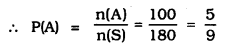(ii) Possibility of one Rs. 5 coin:
n(B) = 180 – 10 = 170
(∵ 10 coins are Rs. 5)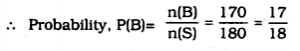Question 11.
Gopi buys a fish from a shop for his aquarium. The shopkeeper taks out one fish at random from a tank containing 5 male fish and 8 female fish (see figure given)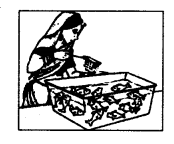What is the probability that the fish taken out is a male fish ?
Solution:
Number of male fish = 5
Number of female fish = 8
∴ Total number of fish, n(S) = 5 + 8 = 13
Probability that the fish taken out is male,
n(E) = 5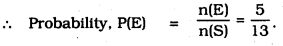Question 12.
A game of chance consists of spinning an arrow which comes to rest pointing at one of the numbers 1, 2, 3, 4, 5, 6, 7, 8 (see the Figure) and these are equally likely outcomes.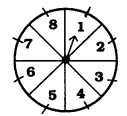What is the probability that it will point at
(i) 8?
(ii) an odd number
(iii) a number greater than 2 ?
(iv) a number less than 9 ?
Solution:
Total numbers in the spinning = 8
∴ n(A) = 1
(i) Possibility that points one number is 8 : n(A) = 1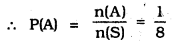(ii) Possibility that points one odd number : 1, 3, 5, 7
∴ n(B) = 4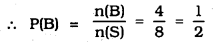(iii) A number greater than 2 :
3, 4, 5, 6, 7, 8
∴ n(C) = 6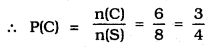(iv) A number less than 9 :
1, 2, 3, 4, 5, 6, 7, 8
∴ n(D) = 8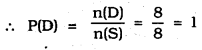Question 13.
A die thrown once. Find the probability of getting
(i) a prime number;
(ii) a number lying between 2 and 6;
(iii) an odd number.
Solution:
Possible event when a die is thrown:
1, 2, 3, 4, 5, 6
∴ n(S) = 6
(i) Probability of getting a Prime number : 2, 3, 5
∴ n(A) = 3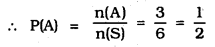(ii) A number lying between 2 and 6 : 3, 4, 5
∴ n(B) = 3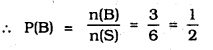(iii) Possibility of odd number : 1, 3, 5
∴ n(C) = 3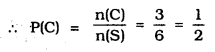Question 14.
One card is drawn from a well-shuffled deck of 52 cards. Find the probability of getting
(i) a king of red colour,
(ii) a face card
(iii) a red face card
(iv) the jack of hearts
(vi) the queen of diamonds.
Solution:
Total number of cards = 52
(i) P(One red king) = 252=126
(ii) Number of a face card :
4 king, 4 queen, 4 jack = Total 12 cards
∴ P(1 face card) = 1252=313
(iii) One red colour face card = 6
∴ P(1 red face card) = 652=326
(iv) P(Heart Jack) = 152
(vi) P(Diamond card) = 152

Question 15.
Five cards— the ten, jack, queen, king and ace of diamonds, are well-shuffled with their face downwards. One card is then picked up at random.
(i) What is the probability that the card is the queen ?
(ii) If the queen is drawn and put aside, what is the probability that the second card picked up is
(a) an ace?
(b) a queen ?
Solution:
Five cards— the ten, jack, queen, king and ace of diamonds.
(i) Probability of getting queen = 15
(ii) If the queen is drawn and put aside, the probability of getting other cards = 4.
(∵ 5 – 1 = 4)
∴ a) Probability that the card is ace = 14
b) Probability that the card is queen = =04=0

Question 16.
12 defective pens are accidentally mixed with 132 good ones. It is not possible to just look at a pen and tell whether or not it is defective. One pen is taken out at random from this lot. Determine the probability that the pen taken out is a good one.
Solution:
Number of defective pens =12
Number of good pens =132
Total Number of pens = 12 + 132 = 144
The probability that the pen taken out is good one,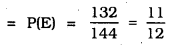Question 17.
(i) A lot of 20 bulbs contain 4 defective ones. One bulb is drawn at random from the lot. What is the probability that the bulb is defective ?
(ii) Suppose the bulb drawn in (i) is not defective and is not replaced. Now one bulb is drawn at random from the rest. What is the probability that this bulb is not defective ?
Solution:
Out of 20 bulbs, contain 4 defective ones.
(i) ∴ Probability of defective bulb = 420
(ii) Out of 20, one bulb is drawn at random from the lot then remaining bulbs = 19.
Out of 19 bulbs, the bulbs which arc not defective = 19 – 4 = 15
∴ The probability that the bulb is not defective, P(E) = 1519

Question 18.
A box contains 90 discs which are numbered from 1 to 90. If one disc is drawn at random from the box, find the probability that it bears
(i) a two-digit number
(ii) a perfect square number
(iii) a number divisible by 5.
Solution:
Number of discs which are numbered from 1 to 30,
n(S) = 90
(i) A two-digit number :
Out of 90, one digit number = 9
∴ 2-digit numbers = 90 – 9 = 81
∴ 2-digit numbers, n(E) = 81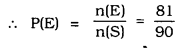(ii) A perfect square number :
1, 4, 9, 16, 25, 36, 49, 64, 81
∴ n(E) = 9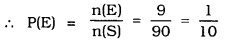(iii) A number divisible by 5 :
5, 10, 15, 20, 25, 30, 35, 40, 45, 50, 55, 60, 65, 70, 75, 80, 85, 90 .
∴ n(E) = 18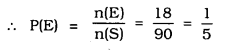Question 19.
A child has a die whose six faces show the letters as given below :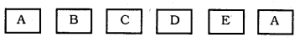The die is thrown once. What is the probbility of getting (i) A ?, (ii) D ?
Solution:
Number of faces in a die is 6.
∴ n(S) = 6
(i) The probability of getting face ‘A’:
∵ n(E) = 2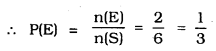(ii) The probbility of getting face ‘D’ is 1.
n(E) = 1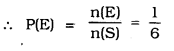Question 20.
Suppose you drop a die at random on the rectangular region as shown in the following figure. What is the probability that it will land inside the circle with diameter 1 m ?
(* Not from the examination point of view)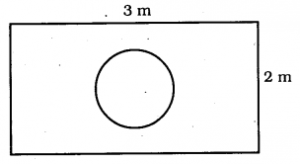Solution:
(i) Length of a rectnagular region is 3m.
Breadth of a rectangular region is 2m.
∴ Area of rectangle = length × breadth
= 3 × 2 = 6 sq.m.
(ii) A circle with diameter 1 m.
∴ Radius, r = 12 m
Area of Circle = πr2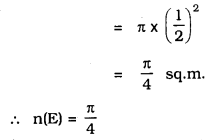∴ The probability that die will land inside the circle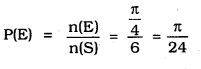Question 21.
A lot consists of 144 ball pens of which 20 are defective arid the others are good. Nuri will buy a pen if it is good, but will not buy If It is defective. The shopkeeper draws one pen at random and gives it to her. What is the probability that
(ii) She will not buy It?
Solution:
Total number of pens, n(S) = 144.
Number of defective pens = 20
∴ Number of good pens = 144 – 20
= 124
(i) Number of good pens Nun buys = 124
∴ n(E) = 124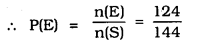(ii) Number of pens which Nuri do not buy = 20
∴ n(E) = 20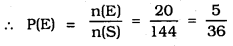Question 22.
Refer to Example 13.
(i) Complete the following table: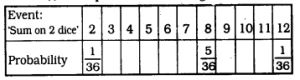(ii) A student argues that there are 11 possible outcomes 2, 3, 4, 5, 6, 7, 8, 9, 10, 11 and 12. Therefore each of them has a probability 111. Do you agree with this argument ? Justify your answer.
Solution:

∵ The two dice are thrown together.
∴ Following are the possible outcomes :
(1, 1) ; (1, 2); (1, 3); (1, 4); (1, 5); (1, 6).
(2, 1) ; (2, 2); (2, 3); (2, 4); (2, 5); (2, 6).
(3, 1); (3, 2); (3, 3); (3, 4); (3, 5); (3, 6).
(4, 1) ; (4, 2); (4, 3); (4, 4); (4, 5); (4, 6).
(5, 1) ; (5, 2); (5, 3); (5, 4); (5, 5); (5, 6).
(6, 1) ; (6,.2); (6, 3); (6, 4); (6, 5); (6, 6).
∴ Total number of possible outcomes is 6 × 6 = 36
(i) (a) The sum on two dice is 3 for (1, 2) and (2, 1)
∴ Number of favourable outcomes = 2
⇒ P(3) = 236

(b) The sum on two dice is 4 for (1, 3), (2, 2) and (3, 1).
∴ Number of favourable outcomes = 3
⇒ P(4) = 336

(c) The sum on two dice is 5 for (1, 4), (2, 3), (3, 2) and (4,1)
∴ Number of favourable outcomes = 4
⇒ P(5) = 536

(d) The sum on two dice is 6 for (1, 5), (2, 4), (3, 3), (4, 2) and (5,1)
∴ Number of favourable outcomes = 5
⇒ P(6) = 536

(e) The sum on two dice is 7 for (1, 6), (2, 5), (3, 4), (4, 3), (5, 2) and (6,1)
∴ Number of favourable outcomes = 6
⇒ P(7) = 6236

(f) The sum on two dice is 9 for (3, 6), (4, 5), (5, 4) and (6, 3)
∴ Number of favourable outcomes = 4
⇒ P(9) = 436

(g) The sum on two dice is 10 for (4, 6), (5, 5), (6,4)
∴ Number of favourable outcomes = 3
⇒ P(10) = 336

(h) The sum on two dice is 11 for (5, 6) and (6,5)
∴ Number of favourable outcomes = 2
⇒ P(11) = 236

Thus, the complete table is as follows: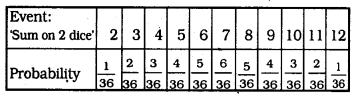(ii) No. The number of all possible outcomes is 36 not 11.
∴ The argument is not correct.

Question 23.
A game consists of tossing a one rupee coin 3 times and noting its outcome each time. Hanif wins if all the tosses give the same result, i.e., three heads or three tails, and loses otherwise. Calculate the probability that Hanif will lose the game.
Solution:
Events when one rupee coin is tossed 3 times :
HHH, TTT, HHT, HTH, HTT, THT, TTH
∴ n(S) = 8
Out of these Event except HHH, TTT
Hanif loosing the game, n(E) = 6
(∵ 8 – 2 = 6)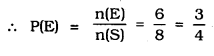Question 24.
A die is thrown twice. What is the probability that
(i) 5 will not come up either Urne?
(ii) 5 will come up at least once?
[Hint: Throwing a die twice and throwing two dice simultaneously are treated as the same experiment.)
Solution:
The possibility that one die is thrown:
n(S) = 6 × 6 = 36
(i) 5 will come up either time:
(1, 5), (2. 5), (3, 5), (4, 5), (5, 5), (6, 5), (5, 1), (5, 2), (5, 3) (5, 4), (5, 6)
∴ Possible events = 11.
∴ Probability that 5 will not come up either time : 36 – 11 = 25
n(E) = 25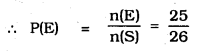(ii) Possibility that 5 will come up at least ones: 11
∴ n(E) = 11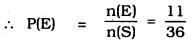Question 25.
Which of the following arguments are correct and which are not correct ? Give reasons for your answer.
(i) If two coins are tossed simultaneously there are three possible outcomes – two heads, two tails or one of each. Therefore, for each of these outcomes, the probability is 13.
(ii) If a die is thrown, there are two possible outcomes — an odd number or an even number. Therefore, the probability of getting an odd number is 12.
Solution:
(i) Given argument is not correct. Because, if two coins are tossed simultaneously then four outcomes are possible (HH, HT, TH, TT). So total outcomes is 4.
∴ The required probability = 14.
(ii) Given argument is correct.
Since, total numebr of possible outcomes = 6
Odd numbers = 3 and even numbers = 3
So, favourable outcomes = 3 (in both the cases even or odd).
∴ Probability = 36=12

All Chapter KSEEB Solutions For Class 10 Maths

—————————————————————————–

All Subject KSEEB Solutions For Class 10

*************************************************

I think you got complete solutions for this chapter. If You have any queries regarding this chapter, please comment on the below section our subject teacher will answer you. We tried our best to give complete solutions so you got good marks in your exam.

If these solutions have helped you, you can also share kseebsolutionsfor.com to your friends.

Best of Luck!!